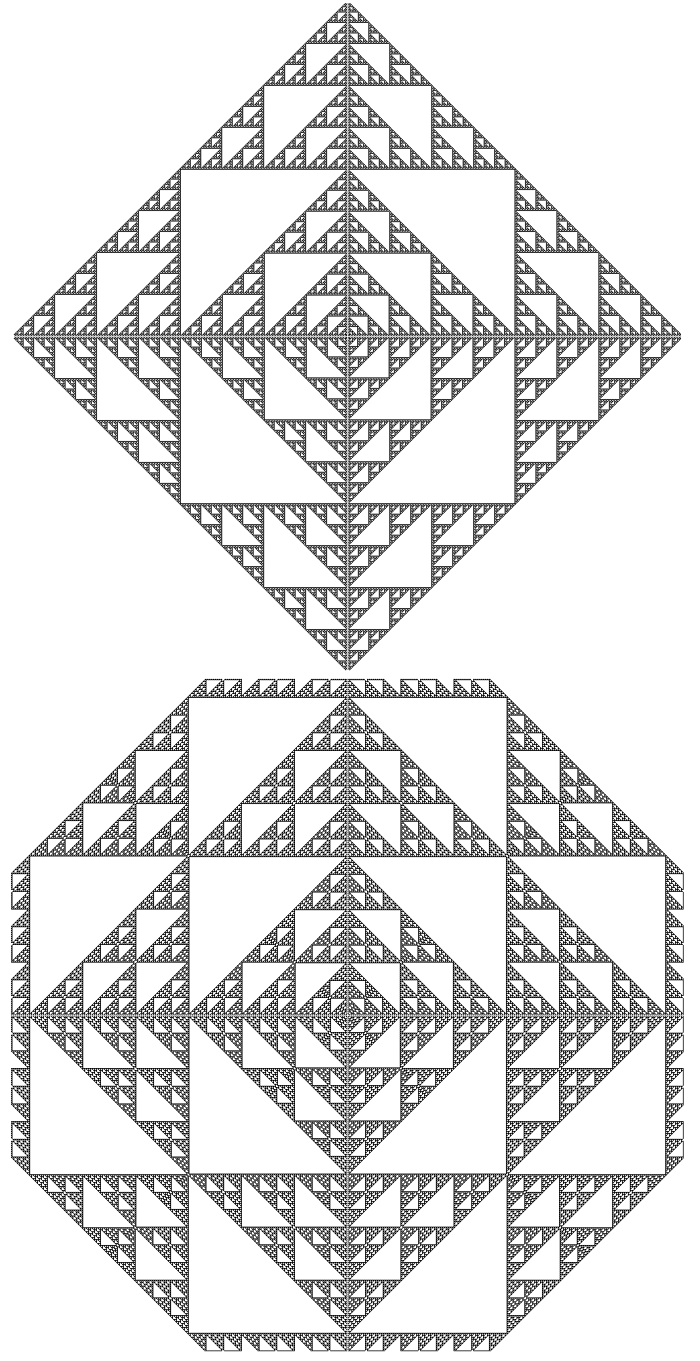# A Second Level Sierpinski from A Pascal's Triangle Sum Modulo Program

By Roger Bagula
Compiled and graphics by Paul Bourke
20 Aug 1998I have been working on the connection between the number theory Pascal's triangle and the Sierpinski set generalizations I was worked out for along time. Last night in the middle of a very hot and uncomfortable night I had an idea. I programmed it up this morning. It works! I don't think anyone else has this.

My generalized Pascal's sum triangles are based on the seed triangle:

1
1 1
1 a 1
1 1+a 1+a 1

Where a is a real number and the recurrence formula is:

1) n(i,j) = Mod(n(i,j - 1) + n(i - 1,j). int(a))

for array n(i,j). This procedure works better than the combination forms based on factorials and binomials because it is always a number that can be calculated with out computer floating point (scientific notation) chopping. I made up a pattern of eight of these to fill the screen symmetrically as a pretty test pattern. The program and one results is part of this article.

I had the idea last night of starting with the seed:

1
1 1
1 a 1

And making the recurrence go one level deeper:

2) n(i,j) = Mod(n(i,j - 1) + n(i - 1,j) + n(i - 1,j - 1), int(a) + 1)

The plus one on the modulo is what works experimentally. The result at a=2 is a Sierpinski carpet version of a Pascal's triangle. This idea is a real number generalization like the first one!

I think that further Sierpinski level generalization equivalents are possible by adding recursive depth as I have done here. The first one that strikes me as symmetrical is:

2) n(i,j) = Mod(n(i,j - 1) + n(i - 1,j) + n(i - 1,j - 2) + n(i - 2,j - 1), b)

where b would be an integer that works for a=2.

These generalizations are real number generalizations of combination theory and represent fundamental orthogonal functional types. The relationship of binary address mapping and Self-similar Hadamard matrices to the Pascal's triangle have been in older articles in TFTN by me. The orthogonality of Hadamard transforms is a practical standard in modern electronics. What we are seeing in Sierpinski space functions is a generalization of orthogonality to a fractal level. Don't let it fool you that I use an experimental mathematics approach: the general application of this mathematics does not depend on who or what by what method the discovery is made.